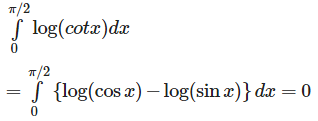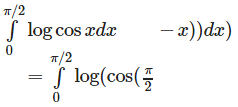# Test: Integrals (CBSE Level) - 2

## 25 Questions MCQ Test Mathematics (Maths) Class 12 | Test: Integrals (CBSE Level) - 2

Description
This mock test of Test: Integrals (CBSE Level) - 2 for JEE helps you for every JEE entrance exam. This contains 25 Multiple Choice Questions for JEE Test: Integrals (CBSE Level) - 2 (mcq) to study with solutions a complete question bank. The solved questions answers in this Test: Integrals (CBSE Level) - 2 quiz give you a good mix of easy questions and tough questions. JEE students definitely take this Test: Integrals (CBSE Level) - 2 exercise for a better result in the exam. You can find other Test: Integrals (CBSE Level) - 2 extra questions, long questions & short questions for JEE on EduRev as well by searching above.
QUESTION: 1

### One value of ∫ f'(x) dx is

Solution:

As (∫f'(x)dx) = f(x) + C, therefore, one value of (∫ f'(x) dx) is f(x)

QUESTION: 2

### ∫ log x dx is equal to

Solution: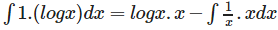QUESTION: 3

###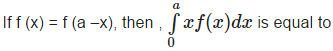Solution: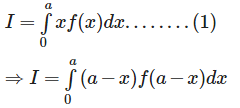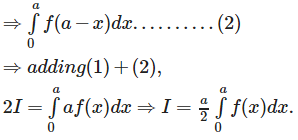QUESTION: 4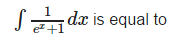Solution:

Solution :- Let e^x/2 = tanθ. Then 1/2e^(x/2)dx = sec^2θdθ.

∫dx/(e^x+1)     = 2∫½ e^(x/2)dx/[e^(x/2)(e^x+1)]

= 2∫[sec^2θdθ]/tanθ(sec^2θ)

= 2∫cosθ/sinθ)dθ = 2ln |sinθ|

From tanθ = e^(x/2) draw a right triangle to see that sinθ = e^x/2√e^x+1:

= 2ln∣e^pi/2√(e^x + 1)|

= ln |e^x/(e^x+1)|

= x−ln(e^x+1)+ C

QUESTION: 5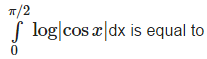Solution: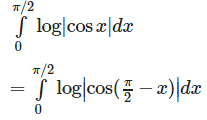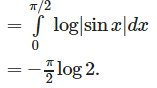QUESTION: 6

if ∫ g(x) dx = g(x), then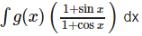is equal to

Solution: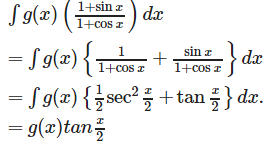QUESTION: 7

If f (x) be a function such that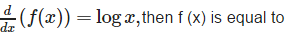Solution:

∫ log xdx = xlog x - x = x(log x -1)

= x(log x - log e) =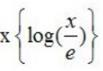QUESTION: 8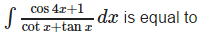Solution: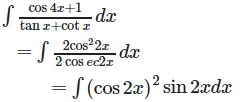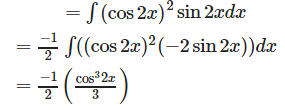QUESTION: 9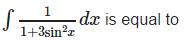Solution:

Dividing numerator and denominator by cos2x and substituting tanx = t , we get :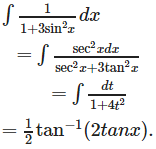QUESTION: 10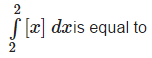Solution: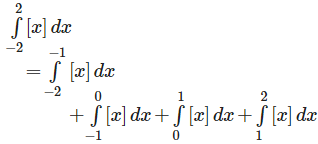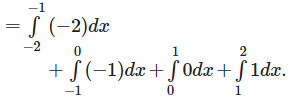QUESTION: 11

∫ sec2 x cosec2 x dx is equal to

Solution: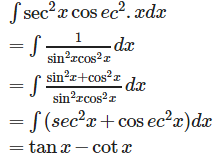QUESTION: 12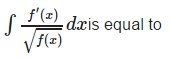Solution: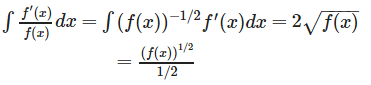QUESTION: 13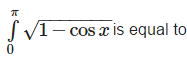Solution: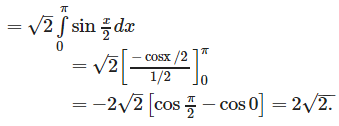QUESTION: 14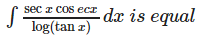Solution: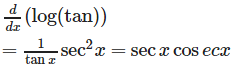QUESTION: 15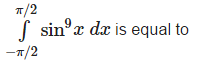Solution:

Note that sin9x is an odd function, therefore,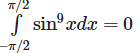QUESTION: 16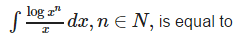Solution: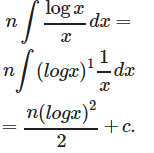QUESTION: 17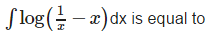Solution:

∫ log(1-x) dx - ∫ log x dx = 0

QUESTION: 18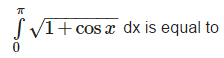Solution: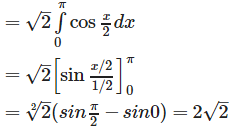QUESTION: 19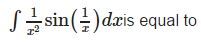Solution: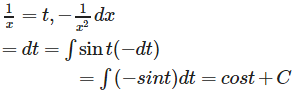QUESTION: 20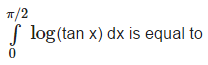Solution: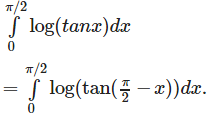QUESTION: 21

∫ (tan x + cot x) dx is equal to

Solution:

Explanation : ∫(tanx + cotx)dx

= ∫tanxdx + ∫cotxdx

= ∫sinx/cosx dx + ∫cosx/sinx dx

I1 = sinx/cosx

Put t = cosx

dt = -sinx dx

I2 = cosx/sinx

Put t = sinx

dt = cosx dx

So, we get

=> ∫-dt/t + ∫dt/t

=> - ln t + ln t + c

=> -ln cosx + ln sinx + c

=> log (sinx/cosx) + c

=> log(tanx)

QUESTION: 22

∫ log(log x) + (log x)-1) dx is equal to

Solution: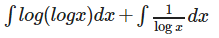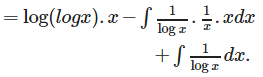QUESTION: 23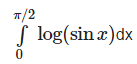Solution: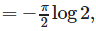QUESTION: 24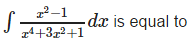Solution: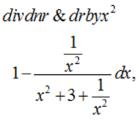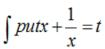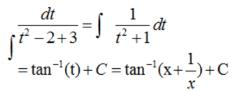QUESTION: 25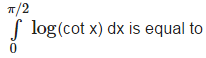Solution: